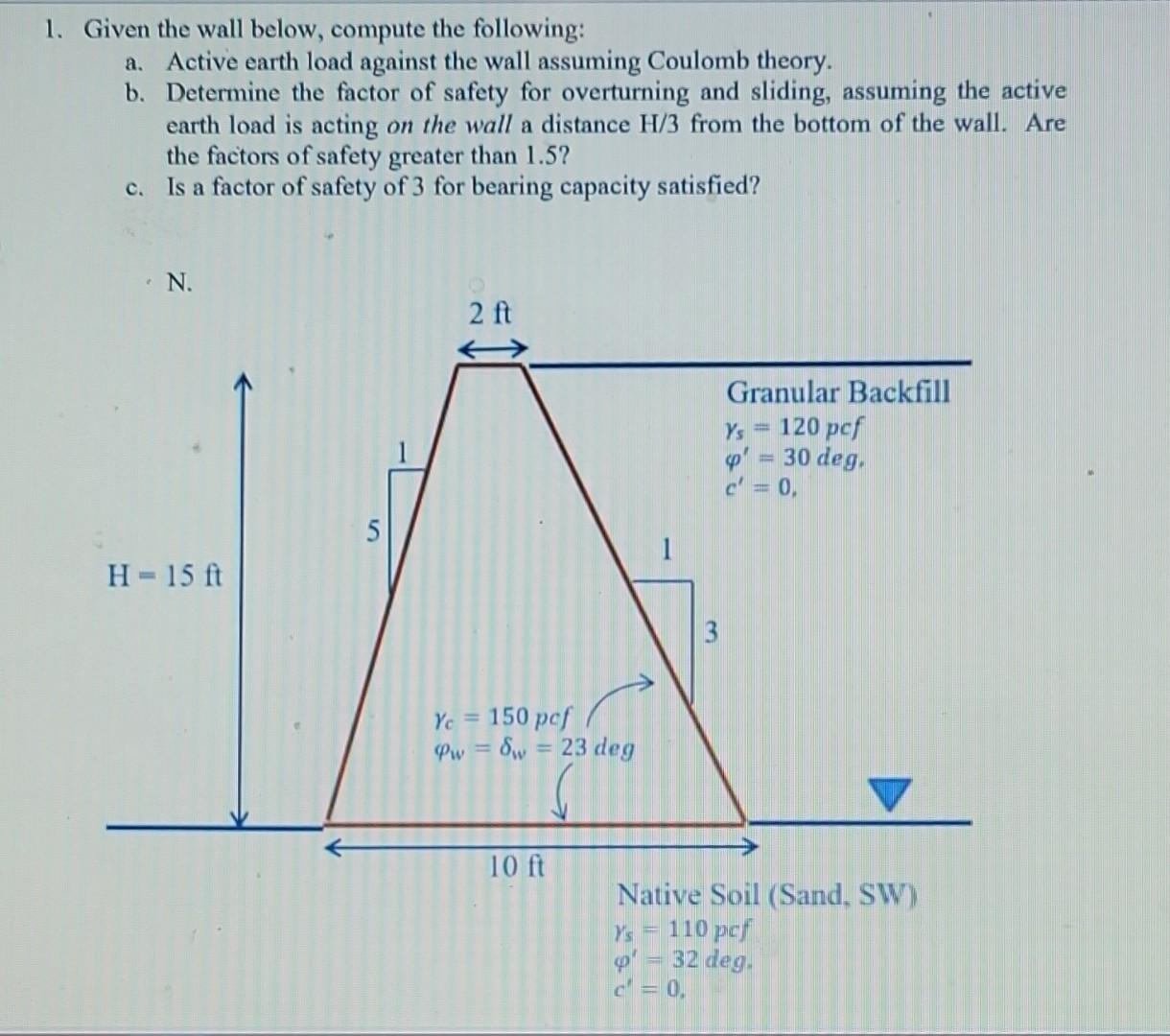# (Solved): 1. Given the wall below, compute the following: a. Active earth load against the wall assuming ...1. Given the wall below, compute the following: a. Active earth load against the wall assuming Coulomb theory. b. Determine the factor of safety for overturning and sliding, assuming the active earth load is acting on the wall a distance from the bottom of the wall. Are the factors of safety greater than 1.5 ? c. Is a factor of safety of 3 for bearing capacity satisfied?

We have an Answer from Expert